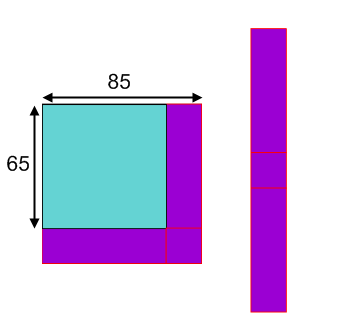#### You may also like### Euler's Squares

Euler found four whole numbers such that the sum of any two of the numbers is a perfect square...### DOTS Division

Take any pair of two digit numbers x=ab and y=cd where, without loss of generality, ab > cd . Form two 4 digit numbers r=abcd and s=cdab and calculate: {r^2 - s^2} /{x^2 - y^2}.### 2-digit Square

A 2-Digit number is squared. When this 2-digit number is reversed and squared, the difference between the squares is also a square. What is the 2-digit number?

# Plus Minus

##### Age 14 to 16Challenge Level

Tom, from Wilson's School, found some other pairs which gave multiples of 1000 and repeated digits:

Other pairs that give multiples of $1000$ are:
$60^2 - 40^2 = 2000$
$70^2 - 30^2 = 4000$
$75^2 - 25^2 = 5000$
$80^2 - 20^2 = 6000$
$85^2 - 15^2 = 7000$
$90^2 - 10^2 = 8000$
All these pairs add up to 100 which is a factor of 1000.
Other ways to make multiples of $1000$ are $95^2 - 45^2 = 7000$ and $95^2 - 55^2 = 6000$. These numbers when added together become a factor of the answer.

Other pairs that give repeated digit answers are:
$67^2 - 34^2 = 3333$
$56^2 - 45^2 = 1111$

The numbers in the tens columns add together to make 9 while the numbers in the units columns add together to make 11.

Tabitha from The Norwood School, Hannah from Munich International School, Richard from Comberton Village College, and Paul, Dulan and Priyan from Wilson's School all explained how the diagram helped them to work out the difference between two square numbers. Here is Richard's explanation:

By drawing a $85 \times 85$ square and a superimposed $65 \times 65$ square, and subtracting the areas, a strip which covers two lengths of the larger square is formed. When segments of the strip are rotated, a rectangle is formed that has a constant width of the difference of the squares' sides and a length of the sum of the squares' sides.So the dimensions of the purple rectangle are: width $85 - 65 = 20$, length $85 + 65 = 150$
This implies that the area of the purple strip is equal to that of the rectangle, $20 \times 150 = 3000$.
In general,by drawing a square of length $x$ with a superimposed square of length $y$, the area of the strip will be $(x+y)(x-y)$, so $x^2 - y^2 = (x+y)(x-y)$

James and Nat from Sawston both used this formula for the difference of two squares to help them to find pairs of numbers. Hannah used the formula to work out some of the other things posed in the problem:

$7778^2 - 2223^2 = (7778 - 2223)(7778+2223) = 5555 \times 10001 = 55555555$
$88889^2 - 11112^2 = (88889 - 11112)(88889 + 11112) = 77777 \times 100001 = 7777777777$

Lyman from Nanjing International School showed how the numbers could be made in lots of different ways by finding factors, including some examples using decimals. Here are some ways of making 1000:

$251^2 - 249^2$
$127^2 - 123^2$
$55^2 - 45^2$
$35^2 - 15^2$
$500.5^2 - 499.5^2$
$102.5^2 - 97.5^2$
$66.5^2 - 58.5^2$
$32.5^2 - 7.5^2$

Finally, Richard explained more generally how to make multiples of 1000 and numbers with repeated digits:

Any number of the form $n \times 10^3$ where $n$ is an integer, can be expressed as $x^2-y^2$ when $x + y = 100$ and $x - y = 10n$.
This is not the only case. More generally, it can be formed when $x + y = u \times 10^a$ and $x - y = v \times 10^b$, where $u \times v < 10$ and $a + b = 3$ or where $u \times v$ is equal to some multiple of $10$ and $a + b = 2$.

This is because $x^2 - y^2 = (x+y)(x-y) = uv \times 10^{(a+b)}$.

A repeated number can also be generated quite easily. A number of the form $mm$ can be expressed as $x^2 - y^2$ when $x + y = 11$ and $x - y$ is equal to $m$.

A number of the form $mnmn$ is expressible when $x + y = 101$ and $x - y = mn$.

$mnomno$ is expressible when $x + y$ is equal to $1001$ and $x - y = mno$

Well done to all who submitted solutions.